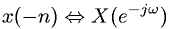Equations > Signal Processing > Fourier Transform Properties > Discrete-Time Fourier time reversal property

### Discrete-Time Fourier time reversal propertyLatex Code:

MathML Code:

 $x\left(-n\right)⇔X\left({e}^{-j\omega }\right)$

MathType 5.0: